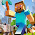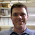### Trend since 1998—significant??

I had a question sent to me about the trend since 1998.  As many of you know, my last post included an analysis which showed that the linear regression trend since 1998 was statistically significant.Trends versus start year.  Error bars are the 95% confidence intervals.
My questioner asked if I had accounted for autocorrelation in my analysis.  The short answer is "No, I did not."  The reason?  According to my analysis, it wasn't necessary.

Here are my methods and R code.

#Get coverage-corrected HadCRUT4 data and rename the first two columns
names(CW)<-"Year"
names(CW)<-"Temp"

#Analysis for autocorrelation—I check manually as well but so far the auto.arima function has performed admirably.
library(forecast)
auto.arima(resid(lm(Temp~Year, data=CW, subset=Year>=1998)), ic=c("bic"))

The surprising result?
Series: resid(lm(Temp ~ Year, data = CW, subset = Year >= 1998))
ARIMA(0,0,0) with zero mean

sigma^2 estimated as 0.005996:  log likelihood=18.23
AIC=-34.46   AICc=-34.18   BIC=-33.69
I was expecting something on the order of ARIMA(1,0,1), which is the autocorrelation model for the monthly averages.  Taking the yearly average rather than the monthly average effectively removed autocorrelation from the temperature data, allowing the use of a white-noise regression model.

trend.98<-lm(Temp~Year, data=CW, subset=Year>=1998)
summary(trend.98)
Call:
lm(formula = Temp ~ Year, data = CW, subset = Year >= 1998)

Residuals:
Min       1Q           Median        3Q          Max
-0.14007  -0.05058   0.01590    0.05696    0.11085

Coefficients:
Estimate       Std. Error    t value    Pr(>|t|)
(Intercept)   -19.405126   9.003395    -2.155     0.0490 *
Year             0.009922      0.004489     2.210     0.0443 *
---
Signif. codes:  0 ‘***’ 0.001 ‘**’ 0.01 ‘*’ 0.05 ‘.’ 0.1 ‘ ’ 1

Residual standard error: 0.08278 on 14 degrees of freedom
Multiple R-squared: 0.2587,    Adjusted R-squared: 0.2057
F-statistic: 4.885 on 1 and 14 DF,  p-value: 0.04425
The other surprise?  That the trend since 1998 was significant even with a white-noise model.  Sixteen data points is not normally enough to reach statistical significance unless a trend is very strong.Temperature trend since 1998

1.Thanks for the additional analysis!

2.This gives me an excellent idea for a parody post.

1.Post the link of the parody when you have write it. I'd love to read it.